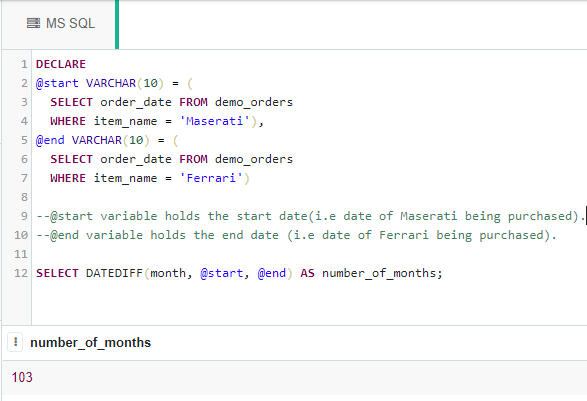# Calculate the Number of Months between two specific dates in SQL

• Last Updated : 26 Apr, 2021

In this article, we will discuss the overview of SQL Query to Calculate the Number of Months between two specific dates and will implement with the help of an example for better understanding. Let’s discuss it step by step.

Overview :
Here we will see, how to calculate the number of months between the two given dates with the help of SQL query using the DATEDIFF() function. For the purpose of demonstration, we will be creating a demo_orders table in a database called “geeks“. There are the following steps to implement SQL Query to Calculate the Number of Months between two specific dates as follows.

Step-1: Creating the Database :
Use the below SQL statement to create a database called geeks as follows.

`CREATE DATABASE geeks;`

Step-2: Using the Database :
Use the below SQL statement to switch the database context to geeks as follows.

`USE geeks;`

Step-3: Table Definition :
We have the following demo table in our geeks database.

```CREATE TABLE demo_orders
(
ORDER_ID INT IDENTITY(1,1) PRIMARY KEY,
--IDENTITY(1,1) is same as AUTO_INCREMENT in MySQL.
--Starts from 1 and increases by 1 with each inserted row.
ITEM_NAME VARCHAR(30) NOT NULL,
ORDER_DATE DATE
);```

Step-4: Verifying :
You can use the below statement to query the description of the created table:

`EXEC SP_COLUMNS demo_orders;`

Output :

Step-5: Adding data to the table :
Use the below statement to add data to the demo_orders table as follows.

```INSERT INTO demo_orders
--no need to mention columns explicitly as we are inserting into all columns and ID gets
--automatically incremented.
VALUES
('Maserati', '2007-10-03'),
('BMW', '2010-07-23'),
('Mercedes Benz', '2012-11-12'),
('Ferrari', '2016-05-09'),
('Lamborghini', '2020-10-20');```

Step-6: Verifying :
To verify the contents of the table use the below statement as follows.

`SELECT * FROM demo_orders;`

Output :

Step-7: SQL Query to Calculate the Number of Months between two specific dates :
Now let’s find the number of months between the dates of an order of ‘Maserati’ and ‘Ferrari’ in the table using the DATEDIFF() function. Below is a syntax for the DATEDIFF() function.

`DATEDIFF(day/month/year, <start_date>, <end_date>);`

Example –

```DECLARE
@start VARCHAR(10) = (
SELECT order_date FROM demo_orders
WHERE item_name = 'Maserati'),
@end VARCHAR(10) = (
SELECT order_date FROM demo_orders
WHERE item_name = 'Ferrari')

--@start variable holds the start date(i.e date of Maserati being purchased).

--@end variable holds the end date (i.e date of Ferrari being purchased).

SELECT DATEDIFF(month, @start, @end) AS number_of_months;

--In place of month we could use year or day and that would give the respective no. of years and
--days in between those dates.```

Output :My Personal Notes arrow_drop_up